# Free Ncert Class 6 Math Algebra Exercise 11.1

Algebra Class 6 Ex. 11.1

## Algebra, Ncert Class 6, Chapter 11, Exercise 11.1 Math Free Solution.

Exercise 11.1

Question 1 :- Find the rule, which give the number of matchsticks required to make the following matchsticks-patterns. Use a variable, to write the rule.
(a) A pattern of letter T as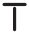(b) A pattern of letter Z as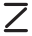(c) A pattern of letter U as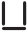(d) A pattern of letter V as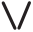(e) A pattern of letter E as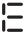(f) A pattern of letter S as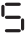(g) A pattern of letter A as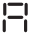Solution 1:-

(a) Number of matchsticks required to make the pattern of= 2n [ as two matchstick used in each letter ]

(b) Number of matchsticks required to make the pattern of= 3n [as three matchstick used in each letter.

(c) Number of matchsticks required to make the pattern of= 3n [as three matchstick used in each letter].

(d) Number of matchsticks required to make the pattern of= 2n [as two matchstick used in each letter].

(e) Number of matchsticks required to make the pattern of= 5n [ as five matchstick used in each letter ]

(f) Number of matchsticks required to make the pattern of= 5n [ as five matchstick used in each letter ]

(g) Number of matchsticks required to make the pattern of= 6n [ as six matchstick used in each letter ]

Question 2 :- We already know, the rule for the pattern of letters L, C and F. Some of the letters from Q.1 (given-above) give us the same-rule as that given by L. Which are these? Why does this happen?

Solution 2:-
Rule for the following letters :-
For L it is 2n
For V it is 2n
For T it is 2n

We observe that the rule is same for L, V and T as because they required only 2 matchsticks.Question 3:- Cadets are marching in a parade. There are 5 cadets in a row. What is the rule, which give the number of cadets, given the number of rows? (use n, for the number of rows.)

Solution 3:-
The number of cadets in a row = 5;
Number of rows = n
Total number of cadets = 5n

Hence, rule is 5n, where n is the number of rows.

Question 4 :- If there are 50 mangoes in a single box, how will you write the total number of mangoes in terms of the number of boxes? (Use b, for the number of boxes.)

Solution 4:-
Number of boxes = b
Number of mangoes in a single box = 50
Total number of mangoes = 50b

Hence, the rule is 50b, where b represents the number of boxes.

Question 5 :- The teacher distributes, 5-pencils per student. Can you tell, how many pencils are needed, given the number of students? (Use s, for the number of students.)

Solution 5:-
Number of students = s
The number of pencils distributed per student = 5;
Total number of pencils required = 5s.

Hence, the rule is 5s, where s represents the number of students.

Question 6  :- A bird flies 1 kilometer in one minute. Can you express, the distance-covered by the bird in terms of its flying time in minutes? (Use t, for flying time in minutes.)

Solution 6:-
The distance covered in 1 minute = 1 km;
The flying time = t
Total distance covered by the bird = t km

Hence, the rule is t km, where t represents the flying time.

Question 7 :- Radha is drawing, a dot-Rangoli (a beautiful-pattern of lines joining-dots) with chalk powder. She has 9 dots in a row. How many dots, will her Rangoli have for r-rows? How many dots, are there if there are 8-rows? If there are 10-rows?

Solution 7:-
Number of rows = r
The number of dots in a row drawn by Radha = 8;
Total number of dots required = 8r

Hence, the rule is 8r, where r represents the number of rows.
For r = 8, the number of dots = (8 x 8) = 64.
For r = 10, the number of dots = (8 x 10) = 80.

Question 8 :- Leela is Radha’s younger sister. Leela is 4 years younger than Radha. Can you write, Leela’s age in terms of Radha’s-age? Take Radha’s age to be x years.

Solution 8:-
Given, Leela’s age = ( Radha’s age – 4 years );
= x years – 4 years
= (x – 4) years.

Question 9 :- Mother has made laddus. She give some laddus, to guests and family members, still 5 laddus remain. If the number of laddus mother gave away is L, how many laddus, did she make?

Solution 9:-
Given that, the number of laddus give-away = L ;
Number of laddus left = 5;

So, the number of laddus made by mother = L + 5.

Question 10 :- Oranges, to be transferred from larger-boxes into smaller-boxes. When large-box is emptied, the oranges from it fill two smaller-boxes and still 10-oranges remain outside. If the number of oranges in a small box are taken to be x, What is the number of oranges in the larger box?

Solution 10:-
Given that, the number of oranges in smaller-box = x ;
Since, the number of oranges in bigger box = 2 x (number of oranges in small box) + (Number of oranges remain outside)
So, the number of oranges in bigger box = ( 2x + 10 ).

Question 11.
(a) Look at the following matchsticks pattern of squares. The squares are not separate. Two neighbouring squares have a common matchstick. Observe the patterns and find the rule which gives the number of matchsticks in terms of the number of squares.
(Hint: If you remove; the vertical-stick at the end, you will get a-pattern of Cs.)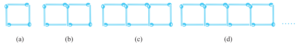…….

(b) Following figure gives, matchstick-pattern of triangles. Find the general rule; that gives the number of matchsticks in terms of the number of triangles.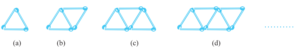Solution 11:-
(a)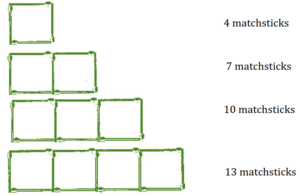If we remove 1 matchsticks from each pattern then they makes table of 3, i.e., 3, 6, 9, 12…

So, the required equation =3x + 1 , where x is number of squares.

(b)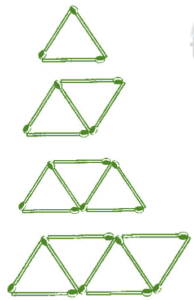If we remove 1 matchsticks from each pattern then they makes table of 2, i.e., 2, 4, 6, 8…

So, the required equation =2x + 1 , where x is number of squares.